# Applied Statistics

### Course description

This course designed to design experiments and carry out statistical analysis of data. Emphasis is given to the development of statistical concepts and statistical computing. Key topics covered include hypothesis testing, simple and multiple linear regression, logistic regression for binary variables and binomial counts, log-linear regression for Poisson counts, and exploratory tools for summarizing multivariate responses, model choice, experimental design, including two-level factorial design, analysis of variance, process control, contingency tables and non-parametric methods. Demonstration of software application is also included in the course.

### Course outcomes

Upon the completion of this course, students will be able to:

• Appreciate and understand the role of statistics
• Develop an ability to apply appropriate statistical methods to summarize and analyze data for some of the more routine experimental settings
• Report the results in appropriate tables or statistical terms
• Perform appropriate statistical techniques
• Interpret results from various statistical software packages

### Course contents

Click the down arrow icon [ 🔽 ] to expand and collapse the course topics.

🔽 1 h 13 min | Special Probability Distributions and Densities
• Concept of probability and their application
• Continuous and discrete probability distribution
• Normal distribution and binomial distribution
• Probabilities of events
🔽 1 h 05 min | Simple Regression
• Concept of estimation of population parameters
• Correlation versus regression
• Simple and multiple linear regression analysis
• Interpret regression coefficients
• Goodness of fit of the model
🔽 1 h 14 min | Multiple Regression
• Simple and multiple linear regressions
• Multiple regression model
• Ordinary Least Square (OLS) method
• Goodness of fit of the regression model
• Functional form of a regression model
🔽 1 h 48 min | Regression Analysis with Limited Dependent Variables
• Economic decisions
• Probit and logit models
• Multinomial logit/probit models
• Ordered logit/probit regression models
🔽 1 h 18 min | Hypothesis Testing
• The null hypothesis and alternative hypothesis
• Outcome of a hypothesis test (p-value)
• One-tail and two-tail tests
• Type-I and II errors and their practical importance in hypothesis testing
• Analysis of Variance (ANOVA)
🔽 1 h 03 min | Experimental Design
• Principles of experimental design
• Classical treatments of design
• The concept of complete randomized design, randomized block design and hierarchical design
• Experimental error

### This course includes: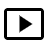7 h 42 min recorded video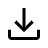Downloadable resources (books and articles)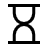Three months access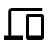Access on mobile and TV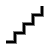Advanced Level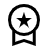Certificate of completion when eligible

#### Self-paced

FREE
3 months of access
This course does not have any sections.